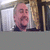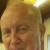## One formula for a single column in Excel 2003Tags:
Microsoft Excel 2003
Microsoft Excel formulas
I am using MS Excel 2003. I have a spreadsheet with the exactly the same formula in every cell of a given column (This is possible by using the row() and the indirect() functions). The spreadsheet is using 6000 rows. Currently, I have copied the formula into each cell. This increases the calculation time of the spreadsheet.

Is it possible to designate a formula for a given column? If so, this would mean I would have only one formula for the whole column and possible speed up the calculation time.

Software/Hardware used:
MS Excel 2003

Thanks. We'll let you know when a new response is added.

## Discuss This Question: 3 Replies

Thanks. We'll let you know when a new response is added.
•You should investigate a macro, that will start at the top and work down to the end of the column, performing the calculation on each cell. The macro can be assigned a button on the work sheet if you want to make it easier for the user, so thy don't need to know the macro name.
report
•Although not exactly what you asked, you might want to look at Tools, Options, Calculations to see if changing it to Manual helps. That way, at least you are choosing when your spreadsheet will be bogged down.
report
•Chippy088's answer is the best. Create a Macro (or VBA script) that runs against the worksheet and calculates all the cells as needed. Then you don't need any formulas in any of the computed cells - just a button to cause the macro to run and compute the values. In Excel Help, look at Macro, VBA, Developer. As an example, the following VBA code will put the row and column numbers in a string of the form "row,column" in the first 6000 rows x 3 columns of a spreadsheet (in much less than one second):
```Sub FillWithJunk
Dim iRow as integer
Dim iCol as Integer
For iRow = 1 to 6000
For iCol = 1 to 3
Worksheets(1).Cells(iRow,iCol).Value = iRow & "," & iCol
Next ' iCol
Next ' iRow
End Sub
```
report